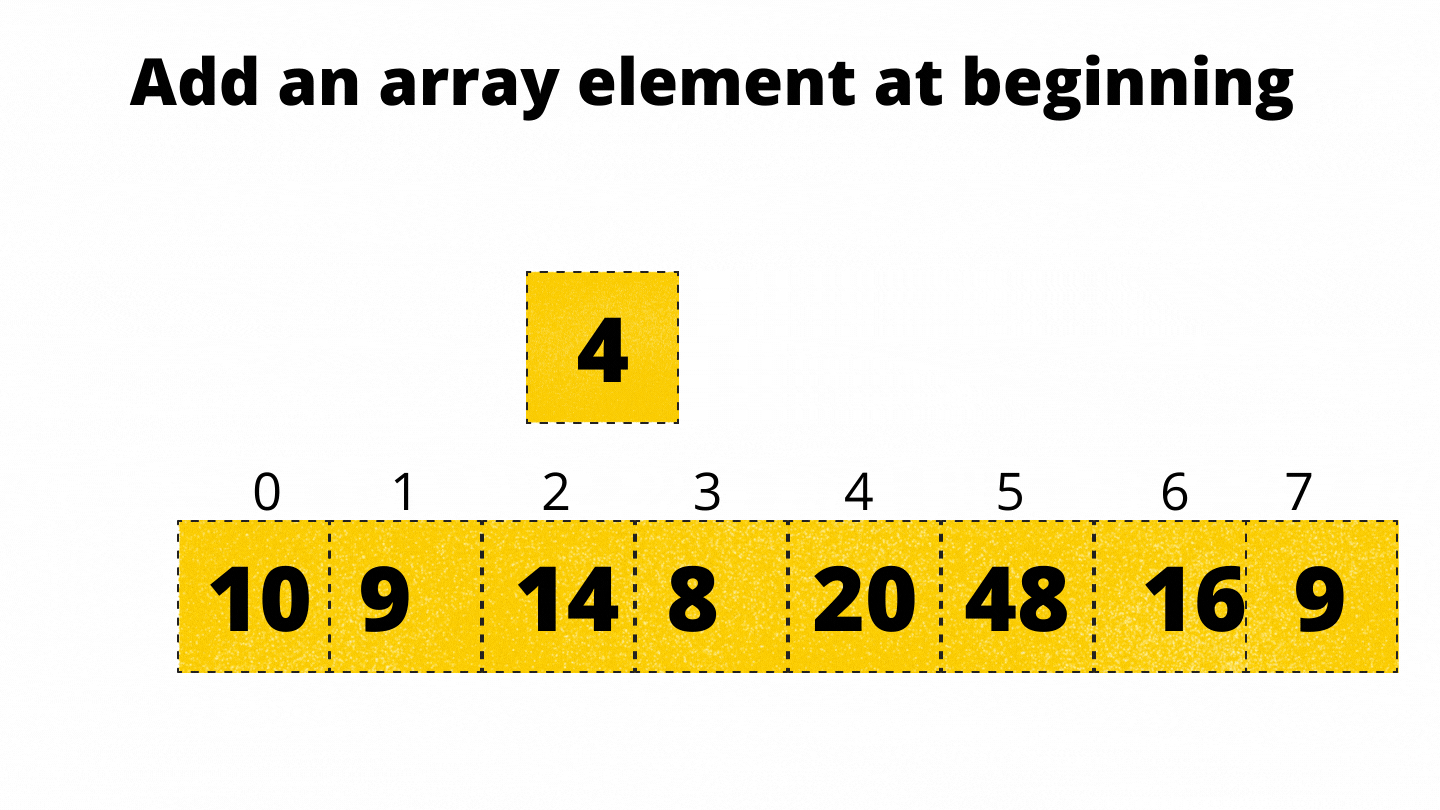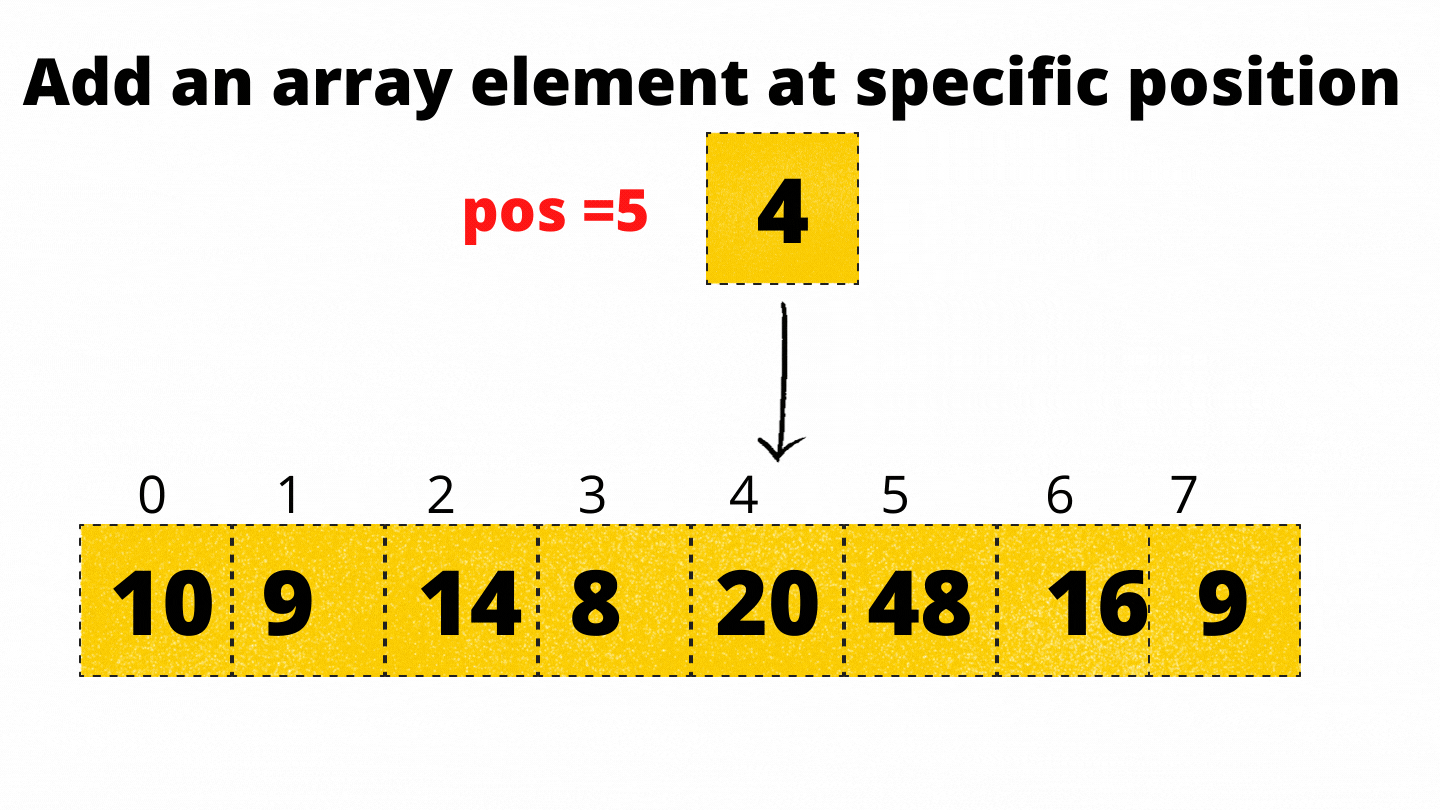# Adding Element in an Array

Problem Statement: Given an array of N integers, write a program to add an array element at the beginning, end, and at a specific position.

```Example:
Input: N = 5, array[] = {1,2,3,4,5}
insertbeginning(6)
insertending(7)
insertatpos(8,4)
Output: 6,1,2,8,3,4,5,7
Explanation: 6 is added at the beginning and 7 is added at the end and 8 is added at position 4 ```

### Solution

Disclaimer: Don’t jump directly to the solution, try it out yourself first.

Approach: The approach is very simple for all three insertions.

• Insertion at beginning

For inserting the element at the beginning we should first shift all elements of the array to left by 1 index and then insert an element at the 0th position.Code:

## C++ Code

``````#include <bits/stdc++.h>
using namespace std;
void insertatbegin(int* arr,int n,int value)
{
for(int i=n-1;i>=0;i--)
{
arr[i+1]=arr[i];
}
arr=value;
}
int main()
{
int n=8;
int arr={10,9,14,8,20,48,16,9};
int value=40;
cout<<"Before inserting the value at beginning:"<<endl;
for(int i=0;i<n;i++)
{
cout<<arr[i]<<" ";
}
cout<<endl;
insertatbegin(arr,n,value);
cout<<"After inserting the value at beginning:"<<endl;
for(int i=0;i<=n;i++)
{
cout<<arr[i]<<" ";
}
cout<<endl;
return 0;
}
``````

Output:

Before inserting the value at beginning:
10 9 14 8 20 48 16 9
After inserting the value at beginning:
40 10 9 14 8 20 48 16 9

Time Complexity: θ(n), since n iterations are required to shift the array element to right by 1 position

Space Complexity: O(1)

## Java Code

``````import java.util.*;
class TUF{
static void insertatbegin(int[] arr,int n,int value)
{
for(int i=n-1;i>=0;i--)
{
arr[i+1]=arr[i];
}
arr=value;
}
public static void main(String args[])
{
int n=8;
int arr[]={10,9,14,8,20,48,16,9,0};
int value=40;
System.out.println("Before inserting the value at beginning:");
for(int i=0;i<n;i++)
{
System.out.print(arr[i]+" ");
}
System.out.println();
insertatbegin(arr,n,value);
System.out.println("After inserting the value at beginning:");
for(int i=0;i<=n;i++)
{
System.out.print(arr[i]+" ");
}
System.out.println();

}
}``````

Output:

Before inserting the value at beginning:
10 9 14 8 20 48 16 9
After inserting the value at beginning:
40 10 9 14 8 20 48 16 9

Time Complexity: θ(n), since n iterations are required to shift the array element to right by 1 position

Space Complexity: O(1)

• Insertion at Ending

For adding the elements at the end, just add the element at the nth index.Code:

## C++ Code

``````#include <bits/stdc++.h>
using namespace std;
void insertatEnd(int *arr, int n, int value)
{
arr[n] = value;
}

int main()
{
int n=8;
int arr={10,9,14,8,20,48,16,9};
int value=40;
cout<<"Before inserting the value at beginning:"<<endl;
for(int i=0;i<n;i++)
{
cout<<arr[i]<<" ";
}
cout<<endl;
insertatEnd(arr,n,value);
cout<<"After inserting the value at beginning:"<<endl;
for(int i=0;i<=n;i++)
{
cout<<arr[i]<<" ";
}
cout<<endl;
return 0;
}
``````

Output:

Before inserting the value at beginning:
10 9 14 8 20 48 16 9
After inserting the value at beginning:
10 9 14 8 20 48 16 9 40

Time Complexity: θ(n), since n iterations are required to shiftθ(1) since we need to directly add an element at the end of the array

Space Complexity: O(1)

## Java Code

``````import java.util.*;
class TUF{
static void insertatEnd(int[] arr, int n, int value)
{
arr[n] = value;
}

public static void main(String args[])
{
int n=8;
int arr[]={10,9,14,8,20,48,16,9,0};
int value=40;
System.out.println("Before inserting the value at beginning:");
for(int i=0;i<n;i++)
{
System.out.print(arr[i]+" ");
}
System.out.println();
insertatEnd(arr,n,value);
System.out.println("After inserting the value at beginning:");
for(int i=0;i<=n;i++)
{
System.out.print(arr[i]+" ");
}
System.out.println();

}
}``````

Output:

Before inserting the value at beginning:
10 9 14 8 20 48 16 9
After inserting the value at beginning:
10 9 14 8 20 48 16 9 40

Time Complexity: θ(n), since n iterations are required to shiftθ(1) since we need to directly add an element at the end of the array

Space Complexity: O(1)

• Insertion at specific position

For adding the element at a specific position, just shift array elements to right by one position, and after that add an element at the desired position.Code:

## C++ Code

``````#include <bits/stdc++.h>
using namespace std;
void insertatposition(int *arr, int n, int value, int pos)
{
for (int i = n; i >= pos; i--)
{
arr[i] = arr[i - 1];
}
arr[pos - 1] = value;
}

int main()
{
int n=8;
int pos = 5;
int arr={10,9,14,8,20,48,16,9};
int value=40;
cout<<"Before inserting the value at beginning:"<<endl;
for(int i=0;i<n;i++)
{
cout<<arr[i]<<" ";
}
cout<<endl;
insertatposition(arr,n,value,pos);
cout<<"After inserting the value at beginning:"<<endl;
for(int i=0;i<=n;i++)
{
cout<<arr[i]<<" ";
}
cout<<endl;
return 0;
}
``````

Output:

Before inserting the value at beginning:
10 9 14 8 20 48 16 9
After inserting the value at beginning:
10 9 14 8 40 20 48 16 9

Time Complexity: O(n) since we need to shift the elements to right according to the position.

Space Complexity: O(1)

## Java Code

``````import java.util.*;
class TUF{
static void insertatposition(int[] arr, int n, int value, int pos)
{
for (int i = n; i >= pos; i--)
{
arr[i] = arr[i - 1];
}
arr[pos - 1] = value;
}

public static void main(String args[])
{
int n=8;
int pos=5;
int arr[]={10,9,14,8,20,48,16,9,0};
int value=40;
System.out.println("Before inserting the value at beginning:");
for(int i=0;i<n;i++)
{
System.out.print(arr[i]+" ");
}
System.out.println();
insertatposition(arr,n,value,pos);
System.out.println("After inserting the value at beginning:");
for(int i=0;i<=n;i++)
{
System.out.print(arr[i]+" ");
}
System.out.println();

}
}``````

Output:

Before inserting the value at beginning:
10 9 14 8 20 48 16 9
After inserting the value at beginning:
10 9 14 8 40 20 48 16 9

Time Complexity: O(n) since we need to shift the elements to right according to the position.

Space Complexity: O(1)

Special thanks to Gurmeet Singh for contributing to this article on takeUforward. If you also wish to share your knowledge with the takeUforward fam, please check out this article